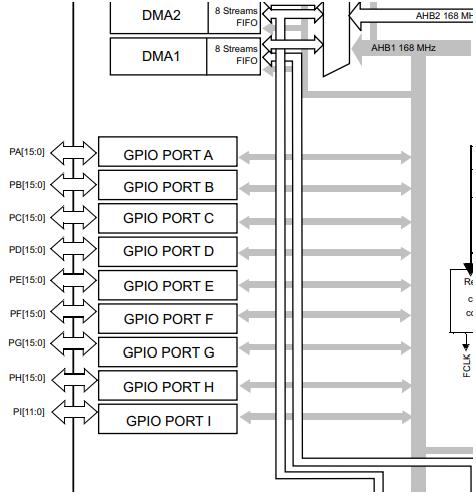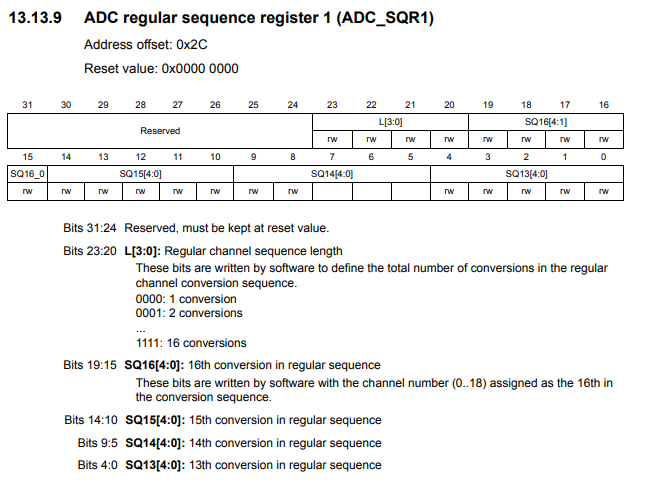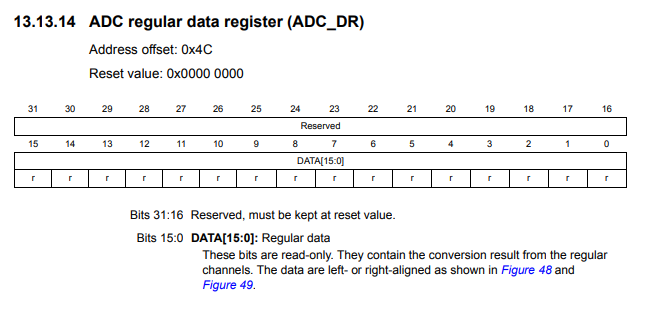﻿ How to Perform Analog to Digital Conversion with an STM32F407G Discovery Board in C ﻿# How to Perform Analog to Digital Conversion with an STM32F407G Discovery Board in CIn this article, we go over how to perform analog to digital conversion with an STM32F407G discovery board in C.

Analog to digital conversion allows us to utilize analog devices in our circuits, such as temperature sensors, potentiometers, etc.

The value that an analog device outputs cannot be read directly by a microcontroller. A microcontroller can only read digital values. Therefore, an ADC is necessary to read the output value of an analog device.

We will show how to sample the ADC using a single conversion mode, which means that we sample the value from the ADC component a single time. We then show how to take continuous samples from the ADC so it continuously gets updated values from the ADC component.

The header file we use that contains needed macros and register structures is shown below.

This file is named stm32f407.h

Its contents are shown below.

This header file contains the needed ADC register structure, as well as other items we need.

### Single Conversion Mode

Below is the main.c file, which contains the functions we need to do an ADC conversion with a single sample from the sensor.

So we have 2 include statements, which includes header files.

We create an integer, adc_value, which is the value returned from the sensor in our circuit.

Within our main() function, we initialize our ADC component with the pa1_adc_init() function.

We then have a while(1) function so that we create an infinite loop (even though it really isn't necessary in this case, since it only samples the ADC component a single time and not continuously).

We start the conversion process with the adc_start_conversion() function.

We read the value into our adc_value variable and then print out the value.

Next, we go to our individual functions.

We have the function, pa1_adc_init(). This function initializes ADC functionality for pin PA1 of our board.

So in order to perform analog to digital conversion with an STM32F407G discovery board, we have to work with a variety of registers.

One of the first things to notice about all of the ADCs on the STM32F407G board is the bus that they are connected to it, which is the APB2 bus. This is important because we have to enable the peripheral clock of this bus in order to use the ADC component attached to it.

This diagram is shown below.You can see that all 3 ADCs are connected to this bus.

Thus, we must enable the clock of this bus.

This is done through the RCC APB2 peripheral clock enable register (RCC_APB2ENR), which is shown below.You can see on this register, that you can activate ADC1 by setting 1 to bit 8 activate ADC2 by setting 1 to bit 9, and activate ADC3 by setting 1 to bit 10.

On this register, you can see all of the rest of the components which are connected to the APB2 bus. This register is how you enable any component attached to this bus.

In our project, we will be working with ADC1, so we set 1 to pin 8.

So, with this now, we have activated the peripheral clock for component ADC1.

Next we need to choose a general I/O pin, which will function as an ADC pin. We need to then activate the peripheral clock register for this GPIO port.

We will choose pin 1 of GPIO port A. This is PA1.

All of the GPIO ports of the STM32F407G board are connected to the AHB1 bus. Therefore, in order to work with a GPIO pin, we need to enable the peripheral clock for the AHB1 bus.

You can see the diagram of this shown below.So the next thing we have to do is look at the AHB1 peripheral clock register (RCC_AHB1ENR). This is shown below.So through this register, we can enable the peripheral clock for any GPIO port. You can see based on the diagram that pin 0 controls GPIO Port A, pin 1 controls GPIO Port B, pin 2 controls GPIO Port C, pin 3 controls GPIO Port D, pin 4 controls GPIO Port E, pin 5 controls GPIO Port F, pin 6 controls GPIO Port G, pin 7 controls GPIO Port I.

In this case, we want to use pin 1 of GPIO Port A, so we enable pin A.

We then must select the mode that the PA1 pin must be in. This is done through the GPIO port mode register (GPIOx_MODER).

This is shown in the diagram below.You can see that in order to make a pin analog, we need to write '11' to the pin. In the case of PA1, we need to write a 11 to pins 2 and 3, which is what we do in our code.

Next, we deal with ADC sampling.

The register we need to deal with in this case is the ADC regular sequence register 3 (ADC_SQR3).

This register is shown in the diagram below.SQ1, the first 4 bits of the register, is the first sequence of the ADC sampling. SQ2 would be if you have another ADC component. SQ3 would be if you have a third ADC component, and so on.

Since we are using ADC1 as the first (the only) sequence, we set bit 0 to 1.

The next thing we need to do is specify the sequence length (how many elements we are doing ADC sampling from).

This is done through the ADC regular sequence register 1. This is shown in the diagram below.Bits 20 to 23 control the regular channel sequence length. This determines the total number of conversions in the regular channel conversion sequence. In our case, it is 1. Therefore, we set bits 20 to 23 to 0. However, since everything should be 0, we simply set the entire register to 0.

The last thing we must do is enable the ADC, ADC1, in our case.

This is done through the ACD control register 2. This is shown in the diagram below.Bit 0 is the ADON bit, which stands for A/D converter ON/OFF. To enable the ADC, a 1 must be set to bit 0.

Next we must on to the next function, adc_start_conversion()

This function actually starts the ADC conversion cycle.

To start the conversion process, we use the ADC control register 2 (ADC_CR2), which is the register shown above .

Bit 30 is the SWSTART bit, which when set to 1 starts the conversion of regular channels.

Before we can get the value of the ADC device, we must wait for the conversion to be complete. We use a while function to check for this. If the conversion is not complete, nothing is done. If the conversion is complete, we return the value from the ADC data register.The value that we get from the ADC regular data register is 12 bits, which means it returns values from 0 o 4095 (4096 data sampling points).

### Continuous Conversions Mode

Note that this is only a single conversion, so it only takes a single sample from the ADC component.

To sample the value from the ADC component continuously, you would have to set the ADC in continuous mode.

Continuous mode is set by setting 1 to bit 1 of ADC control register 2.

So in order to take continuous samples from a sensor, we need to make changes to the main.c file.

The main.c file is shown below.

This places the ADC in continuous sampling mode.

The one change that we must make to the main.c file is that we take the adc_start_conversion() function out of the infinite while loop and place it before this loop.

This is because before, the ADC was in single conversion mode. In single conversion mode, in order to get multiple samples, we must start the conversion process each time (since each time, it only samples a single time).

Howwever, with continuous sampling mode, we only need to start the conversion once. Therefore, we do not want this function in an infinite loop, so that it starts over and over again (which is unnecessary and unwanted when the ADC is in continuous sampling mode). Therefore, we take it out of the infinite while loop and place it before, so that the conversion process is started only once.

The program reads continuous samples from the ADC and prints out these values.

If you want a sample, for example, every second or a certain time period, you would create a delay within the code.

And this is how to perform analog to digital conversion, either a single conversion or continuous conversions, with an STM32F407G discovery board in C.

Related Resources

How to Set Bits of a Number in C

How to Clear Bits of a Number in C